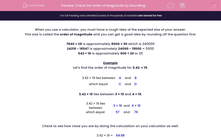# Check the Order of Magnitude by Rounding

In this worksheet, students check the order of magnitude of a calculation by rounding first.This content is premium and exclusive to EdPlace subscribers.Key stage:  KS 2

Curriculum topic:   Maths and Numerical Reasoning

Curriculum subtopic:   Place Value

Difficulty level:#### Worksheet Overview

When you use a calculator, you must have a rough idea of the expected size of your answer.

This size is called the order of magnitude and you can get a good idea by rounding off the question first.

7840 × 28 is approximately 8000 × 30 which is 240000

24216 - 18947 is approximately 24000 - 19000 = 5000

542 ÷ 18 is approximately 500 ÷ 20 or 25

Example

Let's find the order of magnitude for 3.42  × 19.

3.42 × 19 lies between
 A
and
 B
which equal
 C
and
 D

3.42 × 19 lies between 3 × 19 and 4 × 19.

3.42 × 19 lies between
 3 × 19
and
 4 × 19
which equal
 57
and
 76

Check to see how close you are by doing the calculation on your calculator as well.

3.42 × 19 =
 64.98

### What is EdPlace?

We're your National Curriculum aligned online education content provider helping each child succeed in English, maths and science from year 1 to GCSE. With an EdPlace account you’ll be able to track and measure progress, helping each child achieve their best. We build confidence and attainment by personalising each child’s learning at a level that suits them.

Get started